Getting Started with Machine Learning Using TensorFlow and Keras

By ShawnHymel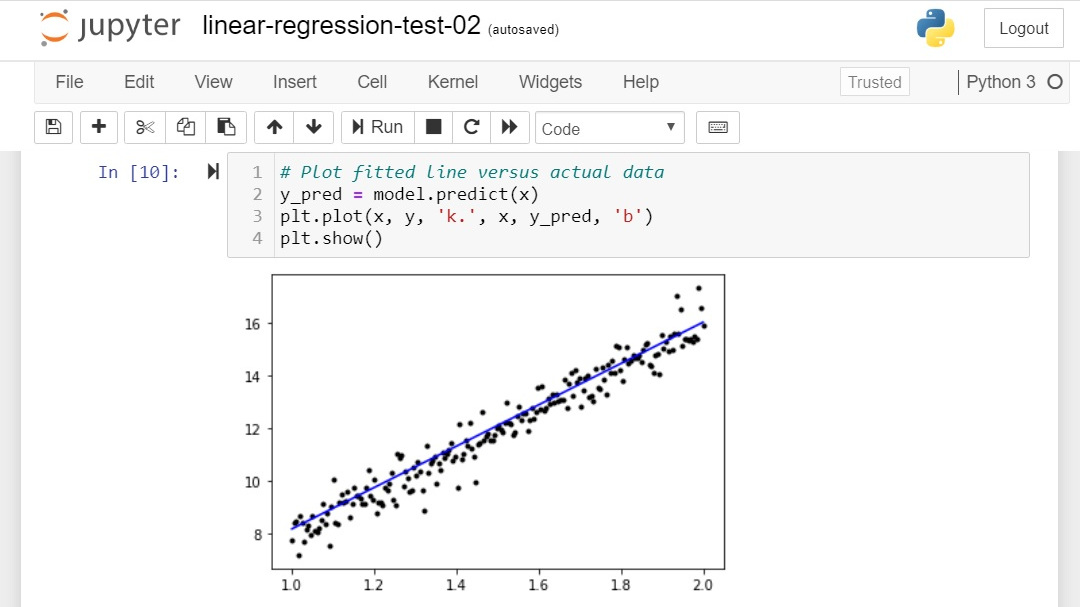The field of Artificial Intelligence (AI) has been around for quite some time. As we move to build an understanding and use cases for Edge AI, we first need to understand some of the popular frameworks for building machine learning models on personal computers (and servers!).

These models can then be deployed to edge devices, such as single-board computers (like the Raspberry Pi) and microcontrollers. To learn more about Edge AI, see this article.

In this tutorial, we show you how to configure TensorFlow with Keras on a computer and build a simple linear regression model. If you have access to a modern NVIDIA graphics card (GPU), you can enable tensorflow-gpu to take advantage of the parallel processing afforded by CUDA.

This information can also be viewed in video format:

Introduction to Keras

Keras is an open source library built for Python that makes training and using deep neural networks much easier. Keras is considered a wrapper layer, as it can be used with a number of different backends, such as TensorFlow and Theano.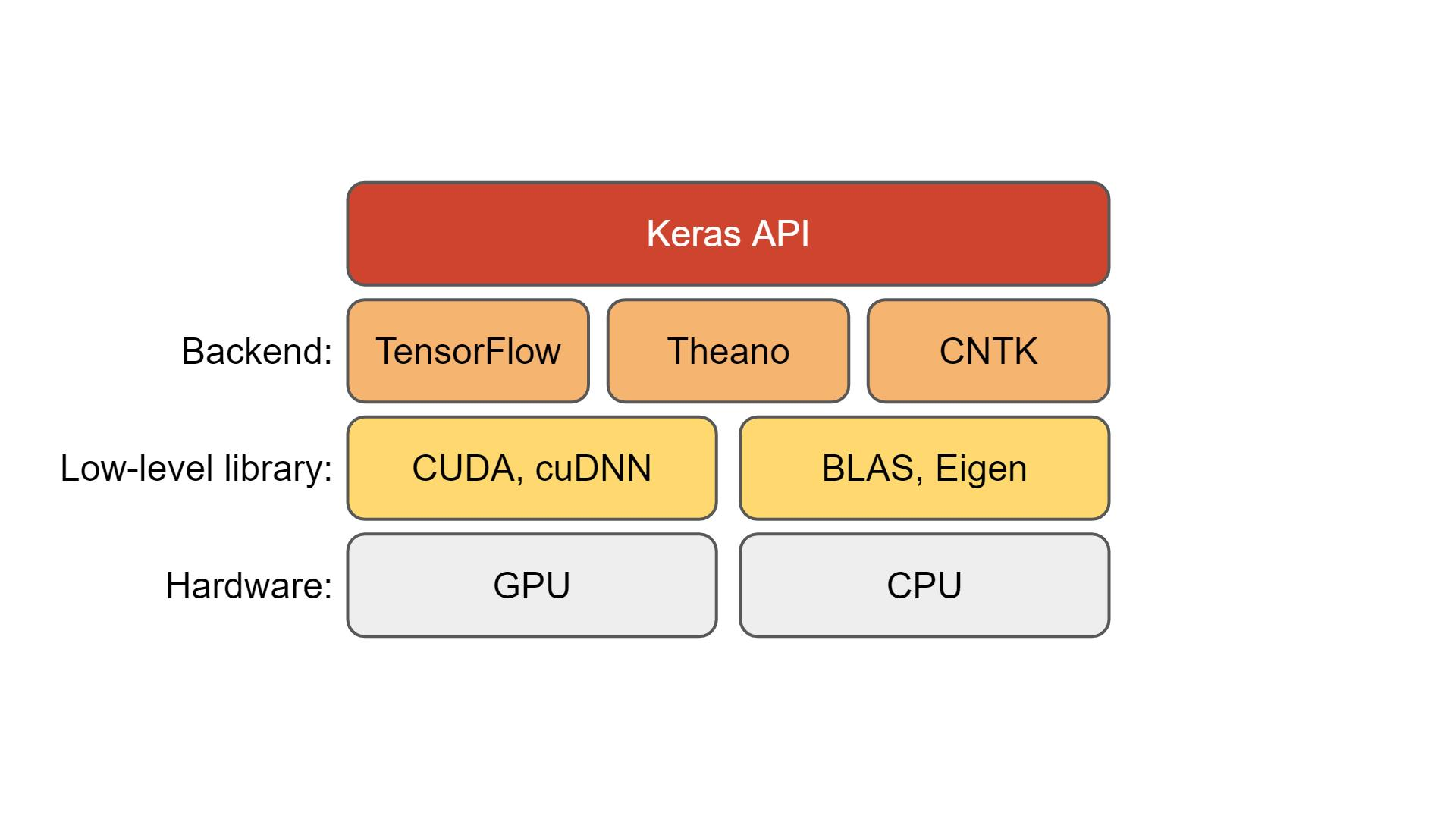Most users find building deep neural networks much easier with Keras, as it wraps up many lines of code from one of these backends into just a few lines. However, note that Keras is intended to be used with neural networks. As such, developing other machine learning algorithms (e.g. support vector machines, regression) is either difficult or not supported in Keras.

On the other hand, the backend frameworks, like TensorFlow, are designed to help users construct all sorts of algorithms. They focus on making things like matrix operations easier, but constructing deep networks can still require many lines of code.

Because TensorFlow is currently the most popular framework for deep learning, we will stick to using it as the backend for Keras. At this time, TensorFlow 2.0 comes bundles with Keras, which makes installation much easier.

Finally, we can use Keras and TensorFlow with either CPU or GPU support. If you have access to an NVIDIA graphics card, you can generally train models much more quickly. This guide will point you to other guides for further instructions on how to install Keras/TensorFlow for the various operating systems with both CPU and GPU support.

Installing TensorFlow and Keras (Linux)

The easiest way to install TensorFlow (which includes Keras) is to use the pre-built Docker image from the TensorFlow website: https://www.tensorflow.org/install/docker. Follow the instructions on that site to download and install the Docker image.

Note that I was unable to test this setup on a Mac, but I assume the steps for using Docker are similar.

There are two important things to note here: first, the image will not work with GPU support out of the box, and second, GPU support is not functional on Windows. If you’re using purely CPU TensorFlow for Linux, using Docker the easiest method.

If you want GPU support on Linux, you will need to perform a few additional steps. I recommend following this guide, which I found to also work on Ubuntu 16.04: https://medium.com/@madmenhitbooker/install-tensorflow-docker-on-ubuntu-18-04-with-gpu-support-ed58046a2a56

Once you have Docker installed and the TensorFlow image downloaded, you can run it with the following command (replace <username> with the username for your system). Note that I’ve created a tf directory in ~/Projects/ to store my TensorFlow project files, and the -v flag maps that directory to the /tf directory in the Docker image.

Copy Code
docker run -it -rm -v /home/<username>/Projects/tf:/tf -p 8888:8888 tensorflow/tensorflow:latest-py3-jupyter

Similarly, if you’d like to enable GPU support (assuming you’ve installed the latest NVIDIA drivers and install nvidia-docker2, as per the link above), you will need to run the following command (once again, replacing <username> with the username on your system):

Copy Code
docker run --runtime=nvidia  -it -rm -v /home/<username>/Projects/tf:/tf -p 8888:8888 tensorflow/tensorflow:latest-gpu-py3-jupyter

This should start a Docker image, which will launch Jupyter Notebook. You should see a link printed in the console. Copy the link, and paste it into a new browser tab.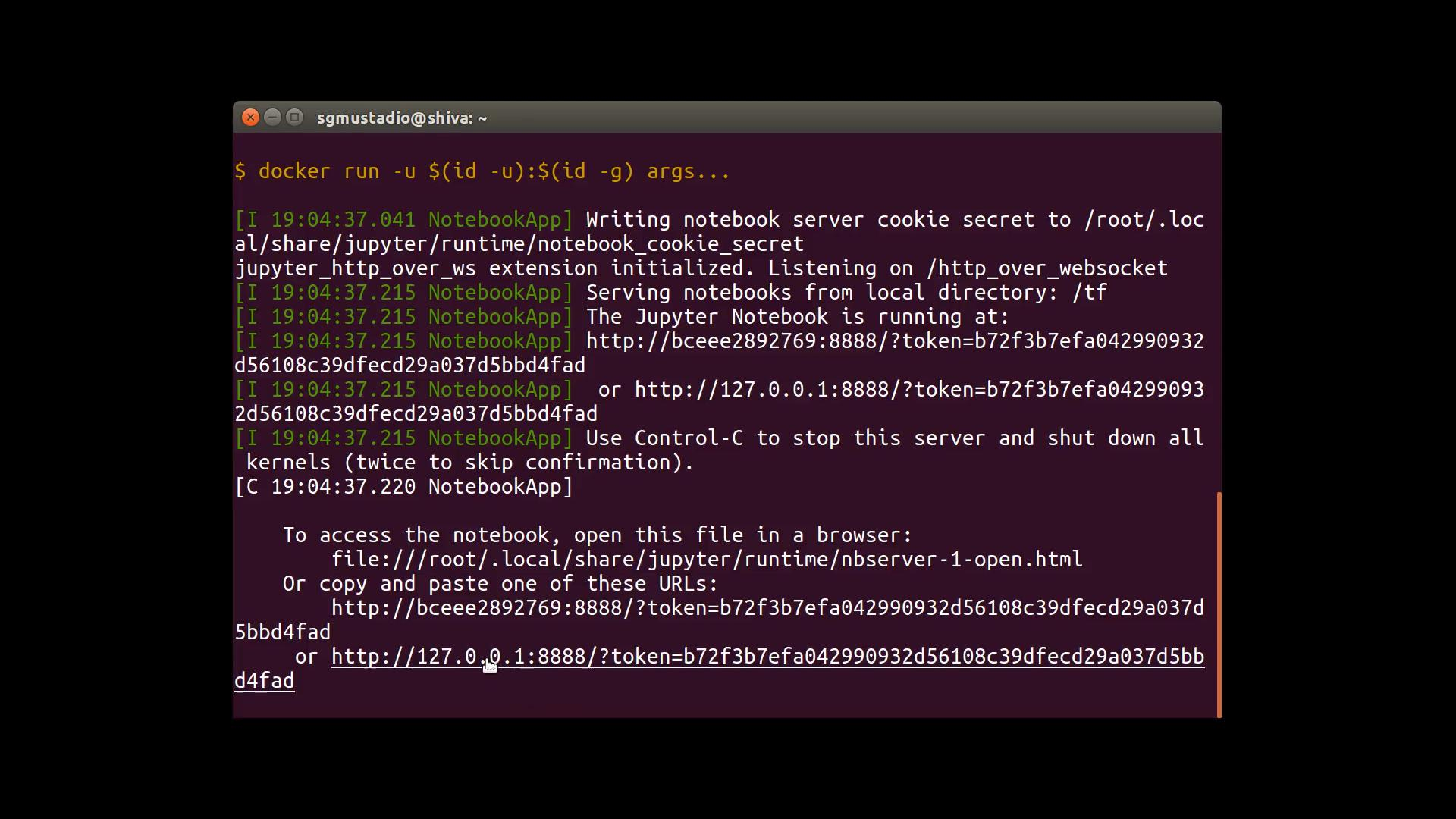If you’d like to see the available tags for the TensorFlow Docker image, see this page: https://hub.docker.com/r/tensorflow/tensorflow/tags/. Note that the order of the tags matters.

Installing TensorFlow and Keras (Windows)

The Docker image for TensorFlow should also work under Windows, but you will not get GPU support at this time. If you’d like GPU support, I recommend installing the NVIDIA CUDA Toolkit, Python, and TensorFlow manually.

For GPU support, you will need to follow the following steps (for just CPU support, skip to the next step of installing Anaconda):

You will then need to install Anaconda, which includes Python and a number of other important libraries and packages. Head to https://www.anaconda.com/distribution/ to download and install Anaconda with Python 3.x (3.7 at the time of this writing) for Windows.

Open the Anaconda Prompt and run the following commands to create a TensorFlow (CPU) environment:

Copy Code
conda create --name tensorflow
conda activate tensorflow
conda install jupyter
conda install scipy
pip install tensorflow

If you wish to also have GPU support (assuming you have an NVIDIA graphics card and followed the steps to install CUDA and cuDNN), also run the following in the Anaconda Prompt:

Copy Code
conda create --name tensorflow-gpu
conda activate tensorflow-gpu
conda install jupyter
conda install scipy
pip install tensorflow-gpu

When you’re ready to run a Jupyter Notebook session with TensorFlow, simply open the Anaconda Prompt and enter the following:

Copy Code
conda activate tensorflow
jupyter notebook

Similarly, if you want to run a Jupyter Notebook session with TensorFlow and GPU support, run the following instead:

Copy Code
conda activate tensorflow-gpu
jupyter notebook

A browser window should automatically open up to the base Jupyter Notebook page. If not, copy the link that appears in the Anaconda Prompt and paste it into a new browser tab.

Testing GPU Support in TensorFlow

To see if we performed all of the installation steps properly to enable GPU support, we can run a simple test. In Jupyter, navigate to a folder where you wish to keep your project files and select New > Python 3.

In a new cell, enter the following code:

Copy Code
import tensorflow as tf
print(tf.test.is_gpu_available())
print(tf.test.is_built_with_cuda())

Run this cell with Cell > Run Cell, the Run button, or by pressing shift+enter. If you have GPU support enabled, both outputs should be True. If you just have CPU support, you will see False printed.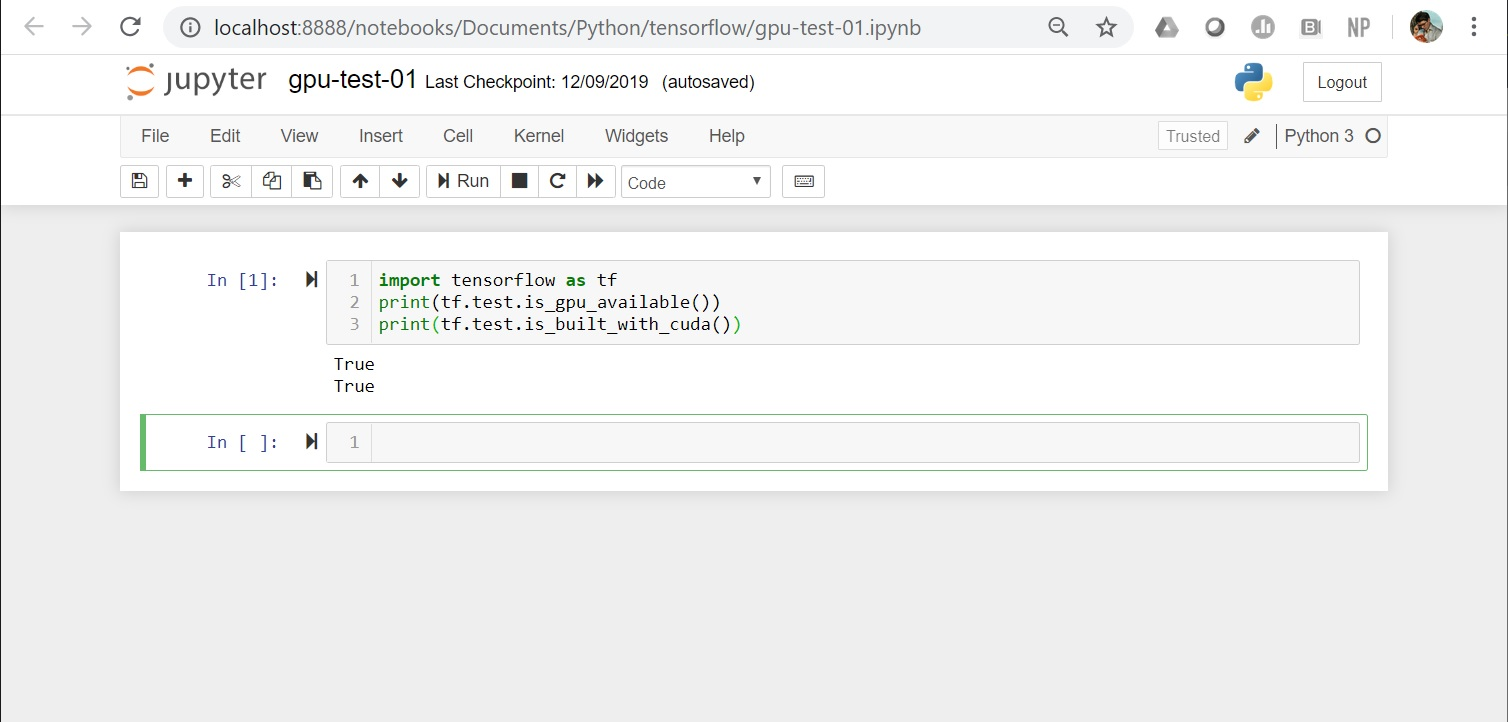Linear Regression with Keras

Linear regression is the process of modeling a relationship between two or more sets of data. In our case, we’re going to create a simple, one-dimensional linear regression model to test TensorFlow and Keras.

We will generate some (mostly) random data and then fit a line to it using stochastic gradient descent (SGD). You don’t need to worry about the details of SGD--Keras will handle it for us!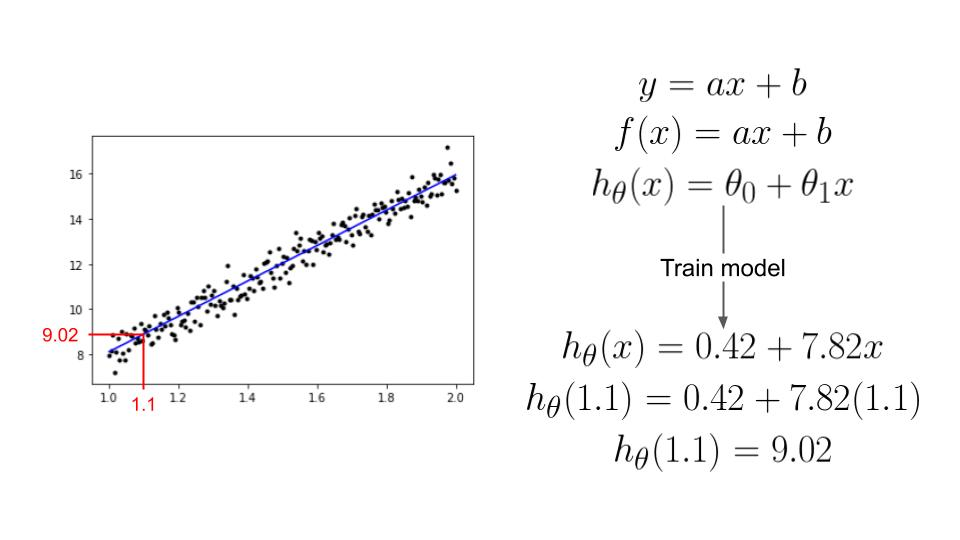You might remember that a line can be represented as y = a*x + b. In machine learning, we often use θ to represent the multipliers (also known as “weights”) for the input data (independent variable(s)), which are given by a and b in the original equation. Instead of using the variable y, we represent the model as the hypothesis function h(x) (just another name for the same equation in this case). We call it the “hypothesis function” because the output attempts to predict some unknown value based on a set of inputs (X).

We use an iterative process of gradient descent (or stochastic gradient descent) to update the unknown weights (θ0, θ1, θ2, etc.) for each data point (a given x and y point in this example). For each x, we calculate the mean square error (MSE) between the output of h(x) for a guessed set of θ weights and the “ground truth” output of y. SGD will give us a new set of θ weights, and we repeat the process. We continue to run this routine over and over again until the MSE is small enough for our liking.

Because Keras works mostly with neural networks, we can use a simple trick to make it work with linear regression. It comes down to understanding how a neural network is constructed. Most neural networks consist of layers of interconnected “nodes.”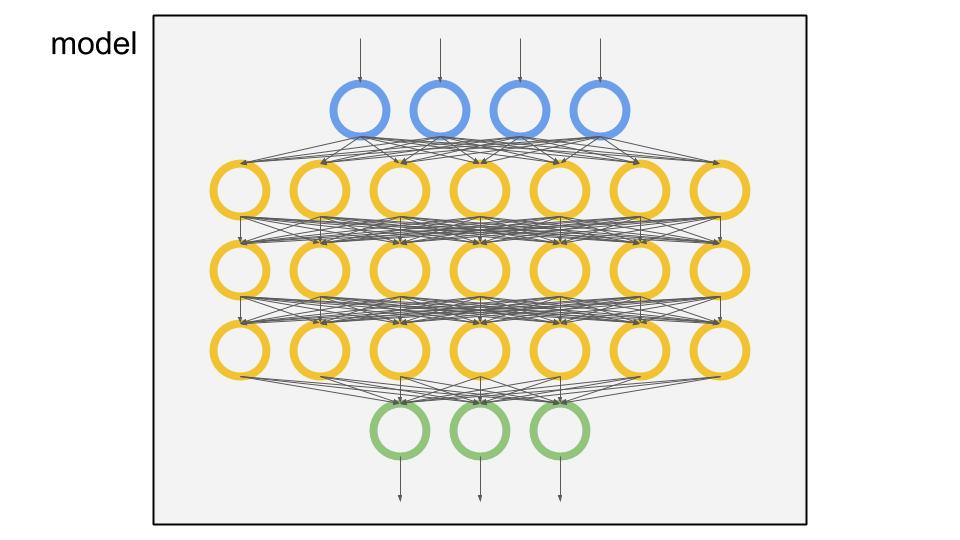What do we get if we create a neural network with just one node?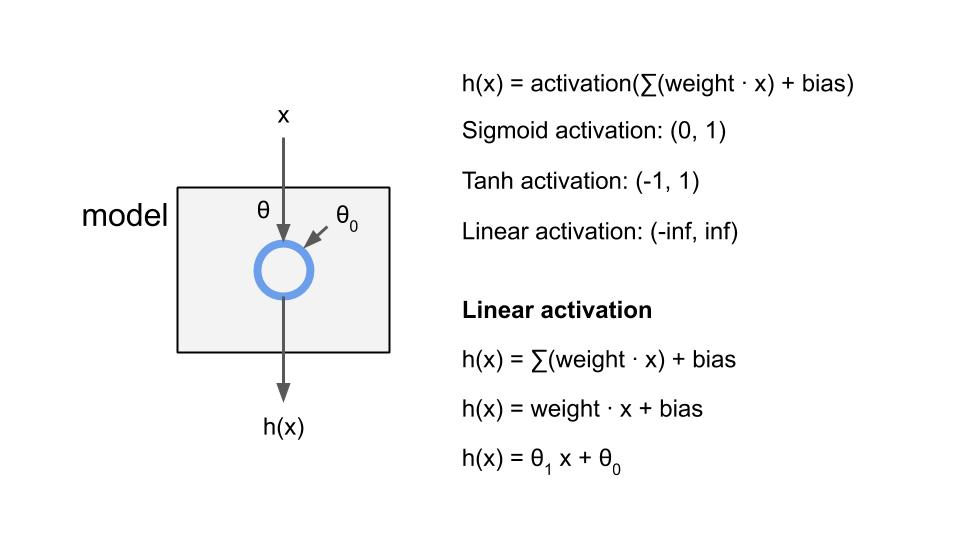Each node in a neural network performs the following mathematical operations on its inputs:

Copy Code
h(x) = activation(∑(θi ∙ xi) + θ0)

It multiplies each input by its associated weight, sums up all those terms, and adds a bias (θ0) term. The output of that is then fed into an “activation” function, which is just a way to constrain the output of the node between a set of values. You’ll generally see activations functions be the sigmoid function or hyperbolic tangent (tanh) function, which re-maps an input real number to some value between 0 and 1 (sigmoid) or -1 and 1 (tanh).

Sometimes (rarely), you’ll see a linear activation function. In our case, we’ll use the linear activation function, which has the effect of multiplying the summation by 1. By doing this, we essentially have no activation function at all, so we just end up with

Copy Code
h(x) = ∑(θi ∙ xi) + θ0

Because we only have 1 input term, we don’t have other θi or xi terms. As a result, we end up with:

Copy Code
h(x) = θ1 ∙ x1 + θ0

This equation looks just like our previous equation for a line! Therefore, we can use all of the built-in Keras functions for training neural networks to create a linear regression model! This might seem like a bit of a hack, but it’s a great way to get familiar with Keras.

Python Code for Training a Linear Regression Model with Keras

Create a new notebook for Python 3. We will need to install matplotlib for our environment. The easiest way to do this is to run the following line in a cell:

Copy Code
!pip install matplotlib

After that’s done, enter the following code into various cells and run them. You can divide them up as you see fit:

Copy Code
import tensorflow.keras
from tensorflow.keras import models, layers, optimizers
import numpy as np

# Create some fake data
x = np.linspace(1, 2, 200)
y = (x * 8) + (np.random.randn(x.shape) * 0.5)

# Examine data
print('x:', x)
print('y:', y)

# Plot the data
import matplotlib.pyplot as plt
plt.plot(x, y, 'k.')
plt.show()

# Build model
model = models.Sequential()

# Get initial, untrained weights
print(model.layers.get_weights())

# Configure training process
model.compile(optimizer=optimizers.SGD(), loss='mse', metrics=['mse'])

# Train model
model.fit(x, y, batch_size=1, epochs=50, shuffle=False)

# Try predicting 1 value
model.predict([1.1])

# Plot fitted line versus actual data
y_pred = model.predict(x)
plt.plot(x, y, 'k.', x, y_pred, 'b')
plt.show()

# Get trained weights
print(model.layers.get_weights())

You should see a graph of your data and the model being trained. Note that batch_size refers to the number of data points we use for each iteration of SGD. One “epoch” occurs when every piece of training data has been used once in the training process. Since we set epochs to 50, then we should see 50 passes of training over all the data.

After the model is done training, we can use it to make predictions. For example, if we want to predict the y value for x=1.1, we can use the function model.predict([1.1]). We should see an output from the model (note that your model might be slightly different, based on the random data generated for training).

We then use matplotlib to draw the line on top of the data to show how well we fit the model.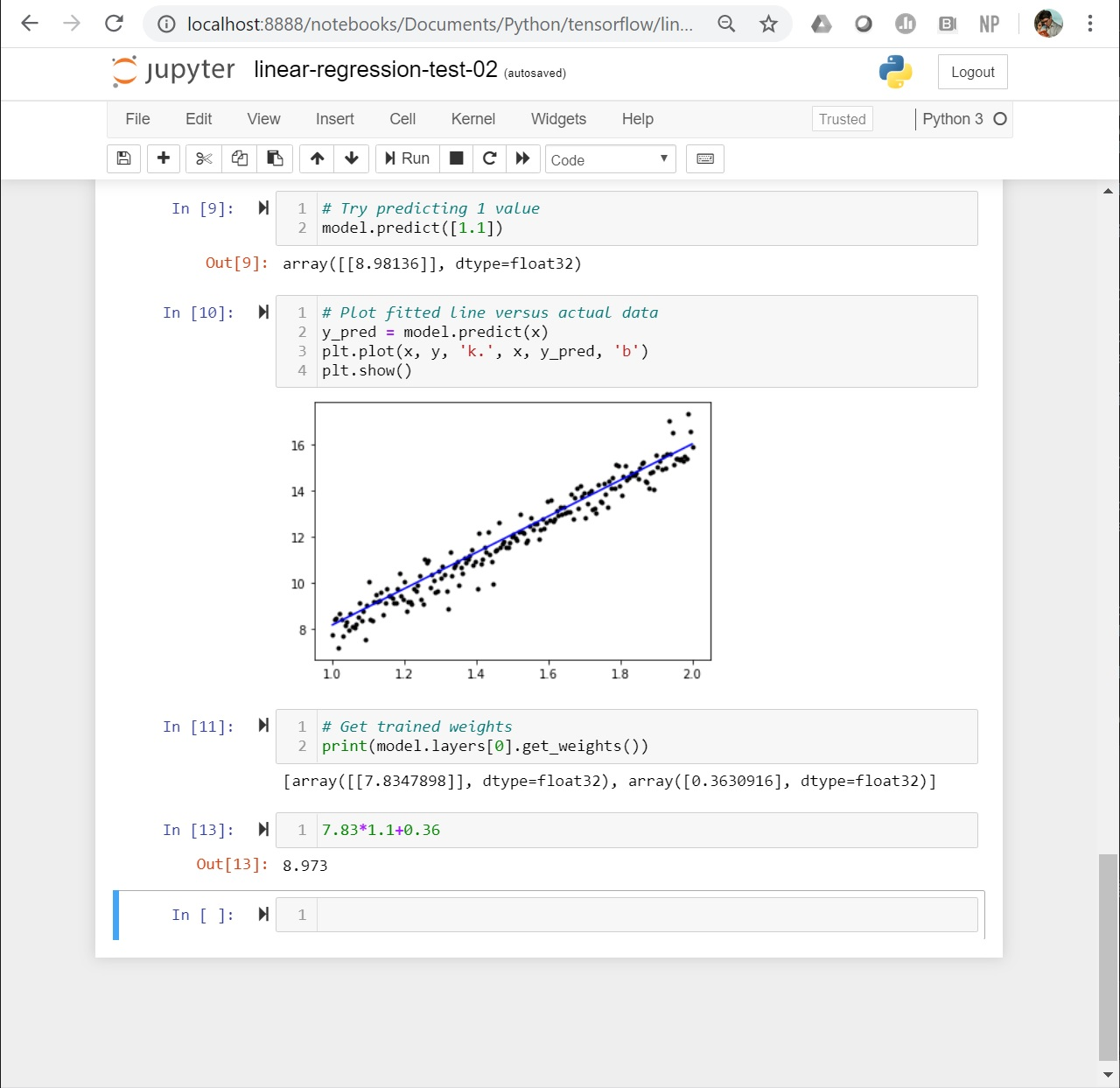Finally, we print out the trained weights of the model with model.layers.get_weights(). In the example above, we can use those weights to manually calculate the y value from a given x=1.1. As you can see, it comes out to be close to the output of model.predict([1.1]).

Wrapping Up

We hope this helps you get started with Keras and TensorFlow! The installation steps can take some time, but once you get your environment set up, using Keras is a breeze. From here, you will need to start understanding when to employ different neural networks to solve different problems, but Keras can save you from having to write tons of code and solve complex equations that make up deep neural networks.